# Symmetric matrix

(diff) ← Older revision | Latest revision (diff) | Newer revision → (diff)
Jump to: navigation, search

A square matrix in which any two elements symmetrically positioned with respect to the main diagonal are equal to each other, that is, a matrixthat is equal to its transpose: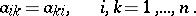A real symmetric matrix of orderhas exactlyreal eigenvalues (counted with multiplicity). Ifis a symmetric matrix, then so are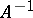and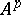, and ifandare symmetric matrices of the same order, then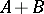is a symmetric matrix, whileis symmetric if and only if.

#### Comments

Every square complex matrix is similar to a symmetric matrix. A real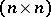-matrix is symmetric if and only if the associated operator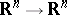(with respect to the standard basis) is self-adjoint (with respect to the standard inner product). A polar decomposition factors a matrixinto a product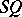of a symmetric and an orthogonal matrix.

Let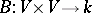be a bilinear form on a vector space(cf. Bilinear mapping). Then the matrix of(with respect to the same basis in the two factors) is symmetric if and only ifis a symmetric bilinear form, i.e.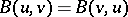.